# Engineering Mechanics - Force System Resultants

Exercise : Force System Resultants - General Questions
• Force System Resultants - General Questions
36.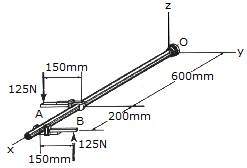Express the moment of the couple acting on the pipe on Cartesian vector form. What is the magnitude of the couple moment?

Mc = (37.5i-25j) N-m, Mc = 45.1 N-m
Mc = (25i-37.5j) N-m, Mc = 45.1 N-m
Mc =45.1kN-m, Mc = 45.1 N-m
Mc =-45.1kN-m, Mc = 45.1 N-m
Explanation:
No answer description is available. Let's discuss.

37.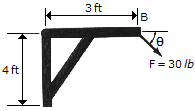Determine the direction (0°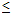180°) of the 30-lb force F so that the moment of F about point A has the maximum magnitude.= 36.9°= 53.1°= 127.0°= 90.0°
Explanation:
No answer description is available. Let's discuss.

38.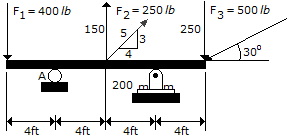Determine the moment of force F2 about point A on the beam.

M2 = 800 ft-lb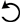M2 = 50 ft-lbM2 = 600 ft-lbM2 = 37.5 ft-lbExplanation:
No answer description is available. Let's discuss.

39.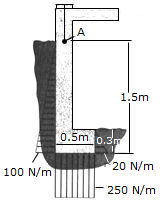The distributed loadings of soil pressure on the sides and bottom of a spread footing are shown. Simplify this system to a single resultant force and couple moment acting at A. What is the resultant force F and the couple moment M?

F = (72i + 125j)N, M = 83.5 N-m CCW
F = (144i + 125j)N, M = 135.7 N-m CCW
F = (144i + 125j)N, M = 83.5 N-m CCW
F = (72i + 125j)N, M = 102.1 N-m CCW
Explanation:
No answer description is available. Let's discuss.

40.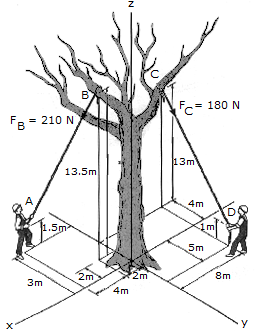Replace the two forces acting on the tree branches by an equivalent force and couple moment acting at point O.

R = (60i+198j-36k) N, M = (747i+18j-924k) N-m
R = (60i+18j-324k) N, M = (747i+18j-924k) N-m
R = (60i+198j-36k) N, M = (360i+1260j-414k) N-m
R = (60i+18j-324k) N, M = (360i+1260j-414k) N-m